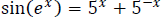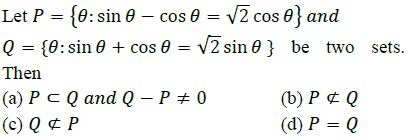## Aspire's Library

A Place for Latest Exam wise Questions, Videos, Previous Year Papers,
Study Stuff for MCA Examinations

## NIMCET Previous Year Questions (PYQs)

#### NIMCET Sets And Relations PYQ

NIMCET PYQ
If A is a subset of B and B is a subset of C, then cardinality of A ∪ B ∪ C is equal to

NIMCET Previous Year PYQNIMCET NIMCET 2020 PYQ

#### Solution

NIMCET PYQ
Let R be reflexive relation on the finite set a having 10 elements and if m is the number of ordered pair in R, then

NIMCET Previous Year PYQNIMCET NIMCET 2023 PYQ

#### Solution

NIMCET PYQ
Number of real solutions of the equationis

NIMCET Previous Year PYQNIMCET NIMCET 2019 PYQ

#### Solution

NIMCET PYQ
Inverse of the function $f(x)=\frac{10^x-10^{-x}}{10^{x}+10^{-x}}$ is

NIMCET Previous Year PYQNIMCET NIMCET 2022 PYQ

#### Solution

Let $f(x)=y$, then

⇒ $\frac{10^x-10^-x}{10^x+10^-x}=y$

⇒ $\frac{10^{2x}-1}{10^{2x}+1}=y$

⇒ $10^{2x}=\frac{1+y}{1-y}$   By Componendo Dividendo Rule

⇒ $x=\frac{1}{2}\log _{10}\Bigg{(}\frac{1+y}{1-y}\Bigg{)}$

⇒ ${f}^{-1}(y)=\frac{1}{2}\log _{10}\Bigg{(}\frac{1+y}{1-y}\Bigg{)}$

⇒ ${f}^{-1}(x)=\frac{1}{2}\log _{10}\Bigg{(}\frac{1+x}{1-x}\Bigg{)}$

NIMCET PYQ
Suppose A1 , A2 , A3 , …..A30 are thirty sets each having 5 elements with no common elements across the sets and B1 , B2 , B3 , ..... , Bn are

NIMCET Previous Year PYQNIMCET NIMCET 2019 PYQ

#### Solution

NIMCET PYQ
A survey is done among a population of 200 people who like either tea or coffee. It is found that 60% of the pop lation like tea and 72% of the population like coffee. Let $x$ be the number of people who like both tea & coffee. Let $m{\leq x\leq n}$, then choose the correct option.

NIMCET Previous Year PYQNIMCET NIMCET 2022 PYQ

#### Solution

NIMCET PYQ
There are two sets A and B with |A| = m and |B| = n. If |P(A)| − |P(B)| = 112 then choose the wrong option (where |A| denotes the cardinality of A, and P(A) denotes the power set of A)

NIMCET Previous Year PYQNIMCET NIMCET 2022 PYQ

#### Solution

NIMCET PYQNIMCET Previous Year PYQNIMCET NIMCET 2018 PYQ

#### Solution

NIMCET PYQ
In a survey where 100 students reported which subject they like, 32 students in total liked Mathematics, 38 students liked Business and 30 students liked Literature. Moreover, 7 students liked both Mathematics and Literature, 10 students liked both Mathematics and Business. 8 students like both Business and Literature, 5 students liked all three subjects. Then the number of people who liked exactly one subject is

NIMCET Previous Year PYQNIMCET NIMCET 2018 PYQ

#### Solution

NIMCET PYQ
If A={1,2,3,4} and B={3,4,5}, then the number of elements in (A∪B)×(A∩B)×(AΔB)

NIMCET Previous Year PYQNIMCET NIMCET 2021 PYQ

#### Solution

NIMCET PYQ
Suppose $A_1,A_2,\ldots,A_{30}$ are 30 sets each with five elements and $B_1,B_2,B_3,\ldots,B_n$ are n sets (each with three elements) such that  $\bigcup ^{30}_{i=1}{{A}}_i={{\bigcup }}^n_{j=1}{{B}}_i=S\,$ and each element of S belongs to exactly ten of the $A_i$'s and exactly 9 of the $B^{\prime}_j$'s. Then $n=$

NIMCET Previous Year PYQNIMCET NIMCET 2021 PYQ

#### Solution

NIMCET PYQ
The number of elements in the power set P(S) of the set S = [2, (1, 4)] is

NIMCET Previous Year PYQNIMCET NIMCET 2017 PYQ

#### Solution

NIMCET PYQ
If X and Y are two sets, then X∩Y ' ∩ (X∪Y) ' is

NIMCET Previous Year PYQNIMCET NIMCET 2021 PYQ

#### Solution

NIMCET PYQ
In a class of 50 students, it was found that 30 students read "Hitava", 35 students read "Hindustan" and 10 read neither. How many students read both: "Hitavad" and "Hindustan" newspapers?

NIMCET Previous Year PYQNIMCET NIMCET 2020 PYQ

#### Solution

P(x)=50,
P(A ∩ B)' = 10
So P(A ∪ B) = 50 - 10 = 40.
So P(A ∩ B) = P(A) + P(B) - P(A ∪ B)
= 30 + 35 - 40 = 25

Solution Contribution by Priyanka Soni

NIMCET PYQ
If A = {4x - 3x - 1 : x ∈ N} and B = {9(x - 1) : x ∈ N}, where N is the set of natural numbers, then

NIMCET Previous Year PYQNIMCET NIMCET 2020 PYQ

#### Solution

A = {0,9,54...}
B = {0,9,18,27...}
So, A ⊂ B

NIMCET PYQ
If A = { x, y, z }, then the number of subsets in powerset of A is

NIMCET Previous Year PYQNIMCET NIMCET 2020 PYQ

#### Solution

NIMCET PYQ
Let A and B be sets. $A\cap X=B\cap X=\phi$ and $A\cup X=B\cup X$ for some set X, relation between A & B

NIMCET Previous Year PYQNIMCET NIMCET 2023 PYQ

#### NIMCET

Online Test Series,
and More.

#### NIMCET

Online Test Series,